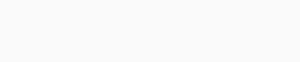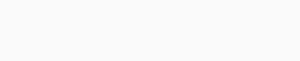During this global pandemic periods we witness, students’ shift to the online learning system. A Mathematics, English and Science competition for students of grades 1 – 12, with six levels. In addition, Art competition for students of grades 1 – 12, with two levels. The key competence tested by FISO online is logical combination, not just pure knowledge of formulas.

Aiming to encourage students to study and critical thinking.  FISO Olympiad team decided to conduct an online Olympiad of FISO Mathematics, English, Science, Chemistry, Biology, Physics and Art Olympiad to boost the motivation of both teachers and students who have been bored, lost goal-oriented character and stimulus for study under this difficult global pandemic times, when most of the Olympiads and competitions are forced to be cancelled or postponed until unknown time. FISO Olympiad team also hopes this competition will help all types of schools to keep their students engaged and to observe their Mathematics, English, Science and Art education in an international arena. (sc : www.fisoolympiad.com)

Berikut ini Soal dan Solusi contoh soal lomba FISO level Grade 7 and 8

1. A bus travel at 90 km/h. How far will it have traveled after 210 minutes?

A) 215 km
B) 235 km
C) 270 km
D) 315 km

\begin{align} Jarak &= 𝐾𝑒𝑐𝑒𝑝𝑎𝑡𝑎𝑛 × 𝑤𝑎𝑘𝑡𝑢\\ &= 90 × \frac{210}{60}\\ &= 315\; 𝑘𝑚\\ \end{align}

2. The sum of the ages of three children is 27. In how many years will the sum of their ages be 63?

A) 10
B) 12
C) 18
D) 36

$$𝑎 + 𝑏 + 𝑐 = 27$$
Jumlah usia mereka $$x$$ tahun kemudian adalah
$$(𝑎 + 𝑥) + (𝑏 + 𝑥) + (𝑐 + 𝑥) = 63$$
$$⇒𝑎 + 𝑏 + 𝑐 + 3𝑥 = 63$$
$$⇒27 + 3𝑥 = 63$$
$$3𝑥 = 63 − 27 = 36$$
$$𝑥 = 12$$
Jadi $$12$$ tahun kemudian jumlah usia mereka bertiga menjadi $$63$$ tahun

3. Jack can wash the family car in 45 minutes. Alex can wash it in 30 minutes. How long will it take them to wash the car if they work together?

A) 18
B) 20
C) 21
D) 22

Gabung kecepatan Jack(J) dan Alex(A)
$$𝐽 + 𝐴 =\frac{1}{45}+\frac{1}{30}=\frac{2 + 3}{90}=\frac{5}{90}=\frac{1}{18}$$
Jadi jika keduanya bekerja sama maka selesai dalam waktu $$18$$ menit

4. Solve

$$\frac{\left[(4)^{-2}:\left(-\frac{1}{3}\right)^2\right]^{\frac{1}{2}}}{\left(-\frac{1}{6}\right)^2} = ?$$

A) – 36
B) – 27
C) 27
D) 48

$$\frac{\left[(4)^{-2}:\left(-\frac{1}{3}\right)^2\right]^{\frac{1}{2}}}{\left(-\frac{1}{6}\right)^2}$$

$$=\frac{\left[(\frac{1}{16}:\left(\frac{1}{9}\right)\right]^{\frac{1}{2}}}{\left(\frac{1}{36}\right)}$$

$$=\frac{\left[(\frac{1}{16}×\left(\frac{9}{1}\right)\right]^{\frac{1}{2}}}{\left(\frac{1}{36}\right)}$$

$$=\frac{\frac{3}{4}}{\frac{1}{36}}$$

$$=\frac{3}{4}×\frac{36}{1}=27$$

5. Solve $$\left[\frac{5}{2}-\frac{1}{1-\frac{1}{2}}\right] : \left[\frac{1}{2}-\frac{\frac{1}{2}}{1+\frac{1}{2}}\right]=?$$

A) 1
B) 1,5
C) 3
D) 3,5

$$\left[\frac{5}{2}-\frac{1}{1-\frac{1}{2}}\right] : \left[\frac{1}{2}-\frac{\frac{1}{2}}{1+\frac{1}{2}}\right]$$

$$=\left[\frac{5}{2}-\frac{1}{\frac{1}{2}}\right] : \left[\frac{1}{2}-\frac{\frac{1}{2}}{\frac{3}{2}}\right]$$

$$=\left[\frac{5}{2}-\frac{2}{1}\right] : \left[\frac{1}{2}-\frac{1}{3}\right]$$

$$=\left[\frac{1}{2}\right] : \left[\frac{1}{6}\right]=3$$

6. Find x?

$$\frac{2x-3}{5}<\frac{x-2}{7}+\frac{1}{3}$$

A) $$x<\frac{27}{68}$$
B) $$x<\frac{68}{27}$$
C) $$x<\frac{27}{50}$$
D) $$x<\frac{50}{27}$$

$$\frac{2𝑥 − 3}{5}<\frac{𝑥 − 2}{7}+\frac{1}{3}$$

$$\frac{2𝑥 − 3}{5}−\frac{𝑥 − 2}{7}<\frac{1}{3}$$

$$\frac{7(2𝑥 − 3)}{35}−\frac{5(𝑥 − 2)}{35}<\frac{1}{3}$$

$$\frac{14𝑥 − 21 − 5𝑥 + 10}{35}<\frac{1}{3}$$

$$\frac{9𝑥 − 11}{35}<\frac{1}{3}$$

$$27𝑥 − 33 < 35$$

$$27𝑥 < 35 + 33 = 68$$

$$𝑥 <\frac{68}{27}$$

7. Add: $$40 + 42 + 44 + … + 94 + 96 =?$$

A) 1960
B) 1964
C) 1970
D) 1972

\begin{align} 40 + 42 + 44 + … + 94 + 96 &=\frac{(40+96)29}{2}\\ &=\frac{136(29)}{2}\\ &=68(29)\\ &=1972\\ \end{align}

8. With the given, find $$a + b + c + d =?$$A) 20
B) 21
C) 22
D) 25Dengan melakukan observasi hasil kali yang mendekati $$4920$$ adalah $$32𝑎 × 15$$,
selanjutnya karena angka satuan dari $$4950$$ adalah maka dipastikan nilai $$𝑎$$ nya adalah genap yaitu $$\{320, 322, 324, 326, 328\}$$, dengan melakukan observasi nilai $$𝑎$$ yang memenuhi adalah $$8$$.
Dari perkalian di atas diperoleh nilai $$b=3, c = 2$$ dan $$d = 8$$
Jadi nilai dari $$𝑎 + 𝑏 + 𝑐 + 𝑑 = 8 + 3 + 2 + 8 = 21$$

9. Solve $$\left|\frac{2𝑥−3}{5}\right|<3$$

A) $$−6<𝑥<9$$
B) $$−6<𝑥<8$$
C) $$−7<𝑥<9$$
D) $$−8<𝑥<10$$

$$−3 <\frac{2𝑥 − 3}{5}< 3$$
$$−15 < 2𝑥 − 3 < 15$$
$$−15 + 3 < 2𝑥 < 15 + 3$$
$$−12 < 2𝑥 < 18$$
$$−6 < 𝑥 < 9$$

10. Find result ofA) $$\frac{9}{20}$$
B) $$\frac{11}{10}$$
C) $$\frac{11}{20}$$
D) $$\frac{10}{11}$$

$$\left(1 −\frac{1}{2^2}\right) \left(1 −\frac{1}{3^2}\right)\left(1 −\frac{1}{4^2}\right) … \left(1 −\frac{1}{10^2}\right)$$
$$= \left(1 −\frac{1}{2}\right)\left(1 +\frac{1}{2}\right)\left(1 −\frac{1}{3}\right)\left(1 +\frac{1}{3}\right)\left(1 −\frac{1}{4}\right)\left(1 +\frac{1}{4}\right) … \left(1 −\frac{1}{10}\right)\left(1 +\frac{1}{10}\right)$$
$$=\left (\frac{1}{2}\right)\left (\frac{3}{2}\right)\left (\frac{2}{3}\right)\left (\frac{4}{3}\right)\left (\frac{3}{4}\right)\left (\frac{5}{4}\right) … \left (\frac{9}{10}\right)\left (\frac{11}{10}\right)$$
$$=\left (\frac{1}{2}\right)\left (\frac{11}{10}\right)$$
$$=\frac{11}{20}$$

11. A bus leaves a city travelling at a speed of 60 km/h. Two hours letter, second bus leaves from the same place, and drives along the same road at 90 km/h. How long will it take for the second bus to catch up with the first bus?A) 2 hours
B) 3 hours
C) 4 hours
D) 5 hoursMisalkan waktu pertemuan dititik $$C$$ adalah $$𝑡$$

$$𝑆_1 = 120 + 𝑆_2$$
$$90𝑡 = 120 + 60𝑡$$
$$90𝑡 − 60𝑡 = 120$$
$$30𝑡 = 120$$
$$𝑡 = 4$$

Jadi kedua mobil bertemu $$4$$ jam kemudian

12. How many ways can a stylist arrange 5 of 8 vases from left to right in a store display?

A) 5720
B) 6720
C) 6270
D) 6770

Banyak cara adalah $$8×7×6×5×4=6720\: cara$$

13. Find $$a + b + c + d$$, if $$\begin{cases} a-b=2\\c-d=4\\a+c=10\end{cases}$$

A) 11
B) 12
C) 13
D) 14

$$𝑎 − 𝑏 + 𝑐 − 𝑑 = 2 + 4 = 6$$
$$⇒𝑎 + 𝑐 − (𝑏 + 𝑑) = 6$$
$$⇒10 − (𝑏 + 𝑑) = 6$$
$$⇒𝑏 + 𝑑 = 4$$
Jadi nilai dari $$𝑎 + 𝑏 + 𝑐 + 𝑑 = (𝑎 + 𝑐) + (𝑏 + 𝑑) = 10 + 4 = 14$$

14. Find $$x?$$

$$\frac{x-1}{2}+\frac{x+1}{4}=2x-\frac{x-1}{4}$$

A) $$-\frac{1}{2}$$
B) $$\frac{1}{2}$$
C) $$-\frac{1}{3}$$
D) $$\frac{1}{3}$$

Kalikan kedua ruas dengan 4

$$4 (\frac{𝑥 − 1}{2}+\frac{𝑥 + 1}{4}) = 4 (2𝑥 −\frac{𝑥 − 1}{4})$$
$$⇒ 2(𝑥 − 1) + (𝑥 + 1) = 8𝑥 − (𝑥 − 1)$$
$$⇒ 2𝑥 − 2 + 𝑥 + 1 = 8𝑥 − 𝑥 + 1$$
$$⇒ 3𝑥 − 1 = 7𝑥 + 1$$
$$⇒ 7𝑥 − 3𝑥 = −1 − 1$$
$$⇒ 4𝑥 = −2$$
$$⇒ 𝑥 = −\frac{2}{4}$$
$$⇒x= −\frac{1}{2}$$

15. Find the surface area ofA) $$196\sqrt 2$$
B) 196
C) $$144+54\sqrt 2$$
D) $$396$$

Panjang sisi miring segitiga alas adalah $$\sqrt{6^2 + 6^2} = \sqrt{2. 6^2} = 6\sqrt 2$$

\begin{align} Luas\; permukaan &= 2𝐿_𝑎 + 𝐾_𝑎 𝑡\\ &= 2 \left(\frac{6×6}{2}\right) + (6 + 6 + 6\sqrt 2)9\\ &= 36 + (12 + 6\sqrt 2)9 = 36 + 108 + 54\sqrt 2\\ &= 144 + 54\sqrt 2\; mm^2\\ \end{align}

16. Find the volume ofA) 86
B) 92
C) 100
D) 104

\begin{align} Volume\; bangun &= Volume\; utuh\; balok\; – \;Volume\; potongan\\ &= 8 × 4 × 4 − 3 × 4 × 2\\ &= 128 – 24\\ &= 104 cm^3\\ \end{align}

17. Find $$x?$$

$$\frac{1-x}{1-\frac{1}{x}}+\frac{x}{1+\frac{1}{x}}-\frac{1}{x+1}=\frac{x-6}{x}$$

A) 9
B) 3
C) 2
D) – 2

$$\frac{1-x}{1-\frac{1}{x}}+\frac{x}{1+\frac{1}{x}}-\frac{1}{x+1}=\frac{x-6}{x}$$

$$⇒\frac{1-x}{\frac{x-1}{x}}+\frac{x}{\frac{x+1}{x}}-\frac{1}{x+1}=\frac{x-6}{x}$$

$$⇒(1-x)(\frac{x}{x-1})+x\frac{x}{x+1}-\frac{1}{x+1}=\frac{x-6}{x}$$

$$⇒-x+\frac{x^2-1}{x+1}=\frac{x-6}{x}$$

$$⇒-x+x-1=\frac{x-6}{x}$$

$$⇒-1=\frac{x-6}{x}$$

$$⇒-x=x-6$$

$$⇒-2x=-6⇒x=3$$

18. Solve

$$\frac{x^2}{2x+1} ÷ \frac{x^2+2x}{2x^2+5x+2}$$

A) 1
B) x
C) x + 2
D) 3x + 1

$$\frac{𝑥^2}{2𝑥 + 1}÷\frac{𝑥^2 + 2𝑥}{2𝑥^2 + 5𝑥 + 2}$$

$$=\frac{𝑥^2}{2𝑥 + 1}×\frac{2𝑥^2 + 5𝑥 + 2}{𝑥^2 + 2𝑥}$$

$$=\frac{𝑥^2}{2𝑥 + 1}×\frac{(𝑥 + 2)(2𝑥 + 1)}{𝑥(𝑥 + 2)}$$

$$= \frac{𝑥^2}{x}=x$$

19. Find $$n=?$$

$$\frac{(n+2)!}{n^3-n)(n-3)!}=6n-12$$

A) 3
B) 4
C) 5
D) 6

$$\frac{(𝑛 + 2)!}{(𝑛^3 − 𝑛) × (𝑛 − 3)!}= 6𝑛 − 12$$

$$⇒\frac{(𝑛 + 2)(𝑛 + 1)𝑛(𝑛 − 1)(𝑛 − 2)(𝑛 − 3)!}{𝑛(𝑛^2 − 1)(𝑛 − 3)!}= 6𝑛 − 12$$

$$⇒\frac{(𝑛 + 2)(𝑛 + 1)𝑛(𝑛 − 1)(𝑛 − 2)(𝑛 − 3)!}{𝑛(𝑛 − 1)(𝑛 + 1)(𝑛 − 3)!}= 6𝑛 − 12$$

$$⇒ (𝑛 + 2)(𝑛 − 2) = 6𝑛 − 12$$

$$⇒ (𝑛 + 2)(𝑛 − 2) = 6(𝑛 − 2)$$

$$⇒ 𝑛 + 2 = 6$$

$$⇒ 𝑛 = 4$$

20. Find the value of x?$$𝑥^{𝑥Δ1} = 81$$
$$⇒𝑥^{𝑥+1} = 81$$
$$⇒3^4 = 81$$
Diperoleh $$𝑥 = 4$$

21. How many tree-digit numbers can be formed from the digits in the number 13567 if a digit cannot be used more than once?

$$Banyak\;bilangan\;adalah = 5×4×3=60\; bilangan$$

22. In how many different ways can six players of a volleyball team be arranged in a line?

$$banyak\; barisan\; berbeda\; adalah\; 6×5×4×3×2×1=720\; cara$$

23. Find the sum of x + y = ?Karena $$Δ𝐴𝐵𝐶 ≈ Δ𝐴𝐵𝐾$$ ,maka perbandingan sisi yang bersesuaian nilainya sama

$$\frac{𝑥 + 12}{3\sqrt 5}=\frac{3\sqrt 5}{𝑥}$$
$$⇒ 𝑥^2 + 12𝑥 = 45$$
$$⇒ 𝑥^2 + 12𝑥 − 45 = 0$$
$$⇒ (𝑥 + 15)(𝑥 − 3) = 0$$
$$⇒ 𝑥 = −15\; 𝑜𝑟\; 𝑥 = 3$$

Nilai $$x$$ yang memenuhi adalah $$3$$
Selanjutnya cari nilai $$y$$ dengan menggunakan rumus pythgaoras
$$𝑦 = \sqrt{(3\sqrt 5)^2 − 3^2} = \sqrt{45 − 9} = \sqrt{36} = 6$$
Jadi nilai $$𝑥 + 𝑦 = 3 + 6 = 9$$

24. In the multiplication and addition tables given, the letters a, b, and c each stand for a positive number. Accordingly, what is the value of b?berdasarkan tabel diperoleh persamaan
$$𝑏𝑐 = 12𝑏$$
$$⟹ 𝑐 = 12$$
Subtitusi nilai $$c$$
$$𝑎 + 𝑏 = 2𝑐 ⇒ 𝑎 + 𝑏 = 24$$
$$𝑏 + 𝑐 = 5𝑎 ⇒ 𝑏 + 12 = 5𝑎$$
Kurangkan kedua persamaan
$$𝑎 − 12 = 24 − 5𝑎$$
$$⇒6𝑎 = 36$$
$$𝑎 = 6$$
Jadi $$𝑏 + 12 = 30 ⇒ 𝑏 = 30 − 12 = 18$$

25. With the given find y = ?$$3 × 4 = 12$$,
$$7 × 12 = 84$$,
$$10 × 84 = 840$$,

jadi $$𝑦 = 13 × 840 = 10920$$